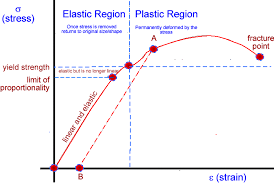# Elasticity

?
• Created by: asda247
• Created on: 20-11-17 10:52

Stress and Strain -Stress is calculated by doing force divided by cross-sectional area, F/A. Measured in Nm-1 and is the force applied per metre squared.

Strain is calculated by doing Change in length divided by original length. Strain has no units and is the ratio of the change in length to the original lengthElastic Limit - is the point where Hooke's law (where stress is propotionate to strain) no longer applies after this point.After this point the material will no longer experience elastic behaviour but instead will behave plastically and won't go back to its original shape once the masses are offloaded.

Plastic deformation - is where the material has passed the point…